# 7 Coordinate Systems, Transformations and Units

## 7.1 Introduction

For all media, the SVG canvas describes "the space where the SVG content is rendered." The canvas is infinite for each dimension of the space, but rendering occurs relative to a finite rectangular region of the canvas. This finite rectangular region is called the SVG viewport. For visual media [CSS2-VISUAL], the SVG viewport is the viewing area where the user sees the SVG content.

The size of the SVG viewport (i.e., its width and height) is determined by a negotiation process (see Establishing the size of the initial viewport) between the SVG document fragment and its parent (real or implicit). Once that negotiation process is completed, the SVG user agent is provided the following information:

• an integer value that represents the width in "pixels" of the viewport
• an integer value that represents the height in "pixels" of the viewport
• (highly desirable but not required) a real number value that indicates how many millimeters a "pixel" represents

Using the above information, the SVG user agent determines the viewport, an initial viewport coordinate system and an initial user coordinate system such that the two coordinates systems are identical. Both coordinates systems are established such that the origin matches the origin of the viewport, and one unit in the initial coordinate system equals one "pixel" in the viewport. (See Initial coordinate system.) The viewport coordinate system is also called viewport space and the user coordinate system is also called user space.

Lengths in SVG can be specified as:

• (if no unit identifier is provided) values in user space -- for example, "15"
• (if a unit identifier is provided) a length expressed as an absolute or relative unit measure -- for example, "15mm" or "5em"

The supported length unit identifiers are: em, ex, px, pt, pc, cm, mm, in, and percentages.

A new user space (i.e., a new current coordinate system) can be established at any place within an SVG document fragment by specifying transformations in the form of transformation matrices or simple transformation operations such as rotation, skewing, scaling and translation. Establishing new user spaces via coordinate system transformations are fundamental operations to 2D graphics and represent the usual method of controlling the size, position, rotation and skew of graphic objects.

New viewports also can be established. By establishing a new viewport, you can redefine the meaning of some of the various unit identifiers (px, pt, pc, cm, mm, in, and percentages) and provide a new reference rectangle for "fitting" a graphic into a particular rectangular area. ("Fit" means that a given graphic is transformed in such a way that its bounding box in user space aligns exactly with the edges of a given viewport.)

## 7.2 The initial viewport

The SVG user agent negotiates with its parent user agent to determine the viewport into which the SVG user agent can render the document. In some circumstances, SVG content will be embedded in or referenced by a containing document. This containing document might include attributes, properties and/or other parameters (explicit or implicit) which specify or provide hints about the dimensions of the viewport for the SVG content. SVG content itself is required to specify information about the appropriate viewport region for the content via the width and height XML attributes that are required on every 'svg' element. The negotiation process uses any information provided by the containing document and the SVG content itself to choose the viewport location and size.

When the SVG content is embedded inline within a containing document, and that document is styled using CSS, then if there are CSS [CSS2] positioning properties [CSS2-POSN] specified on the outermost 'svg' element that are sufficient to establish the width of the viewport, then these positioning properties establish the viewport's width; otherwise, the width attribute on the outermost 'svg' element establishes the viewport's width. Similarly, if there are CSS [CSS2] positioning properties [CSS2-POSN] specified on the outermost 'svg' element that are sufficient to establish the height of the viewport, then these positioning properties establish the viewport's height; otherwise, the height attribute on the outermost 'svg' element establishes the viewport's height.

If the width or height attributes on the outermost 'svg' element are in user units (i.e., no unit identifier has been provided), then the value is assumed to be equivalent to the same number of "px" units (see Units).

In the following example, an SVG graphic is embedded within a parent XML document which is formatted using CSS layout rules. Since CSS positioning properties are not provided on the outermost 'svg' element, the width="100px" and height="200px" attributes determine the size of the initial viewport:

```<?xml version="1.0" standalone="yes"?>
<parent xmlns="http://some.url">

<!-- SVG graphic -->
<svg xmlns='http://www.w3.org/2000/svg'
width="100px" height="200px">
<path d="M100,100 Q200,400,300,100"/>
<!-- rest of SVG graphic would go here -->
</svg>

</parent>
```

The initial clipping path for the SVG document fragment is established according to the rules described in The initial clipping path.

## 7.3 The initial coordinate system

For the outermost 'svg' element, the SVG user agent determines an initial viewport coordinate system and an initial user coordinate system such that the two coordinates systems are identical. The origin of both coordinate systems is at the origin of the viewport, and one unit in the initial coordinate system equals one "pixel" in the viewport. In most cases, such as stand-alone SVG documents or SVG document fragments embedded within XML parent documents where the parent's layout is determined by CSS [CSS2] or XSL [XSL], the initial viewport coordinate system (and therefore the initial user coordinate system) has its origin at the top/left of the viewport, with the positive x-axis pointing towards the right, the positive Y axis pointing down, and text rendered with an "upright" orientation, which means glyphs are oriented such that Roman characters and full-size ideographic characters for Asian scripts have the top edge of the corresponding glyphs oriented upwards and the right edge of the corresponding glyphs oriented to the right.

Example InitialCoords below shows that the initial coordinate system has the origin at the top/left with the x-axis pointing to the right and the y-axis pointing down. The initial user coordinate system has one user unit equal to the parent (implicit or explicit) user agent's "pixel".

```<?xml version="1.0" standalone="no"?>
<!DOCTYPE svg PUBLIC "-//W3C//DTD SVG 20000802//EN"
"http://www.w3.org/TR/2000/CR-SVG-20000802/DTD/svg-20000802.dtd">
<svg width="300px" height="100px">
<desc>Example InitialCoords - SVG's initial coordinate system</desc>

<g style="fill:none; stroke:black; stroke-width:3">
<line x1="0" y1="1.5" x2="300" y2="1.5" />
<line x1="1.5" y1="0" x2="1.5" y2="100" />
</g>
<g style="fill:red; stroke:none">
<rect x="0" y="0" width="3" height="3" />
<rect x="297" y="0" width="3" height="3" />
<rect x="0" y="97" width="3" height="3" />
</g>
<g style="font-size:14 font-family:Verdana">
<text x="10" y="20">(0,0)</text>
<text x="240" y="20">(300,0)</text>
<text x="10" y="90">(0,100)</text>
</g>
</svg>
```## 7.4 Coordinate system transformations

A new user space (i.e., a new current coordinate system) can be established by specifying transformations in the form of a transform attribute on a container element or graphics element. The transform attribute transforms all user space coordinates and lengths on the given element and all of its ancestors. Transformations can be nested, in which case the effect of the transformations are cumulative.

The following demonstrates simple transformations:

Example OrigCoordSys below shows a document without transformations. The text string is specified in the initial coordinate system.

```<?xml version="1.0" standalone="no"?>
<!DOCTYPE svg PUBLIC "-//W3C//DTD SVG 20000802//EN"
"http://www.w3.org/TR/2000/CR-SVG-20000802/DTD/svg-20000802.dtd">
<svg width="400px" height="150px">
<desc>Example OrigCoordSys - Simple transformations: original picture</desc>
<g style="fill:none; stroke:black; stroke-width:3">
<!-- Draw the axes of the original coordinate system -->
<line x1="0" y1="1.5" x2="400" y2="1.5" />
<line x1="1.5" y1="0" x2="1.5" y2="150" />
</g>
<g>
<text x="30" y="30" style="font-size:20 font-family:Verdana">
ABC (orig coord system)
</text>
</g>
</svg>
```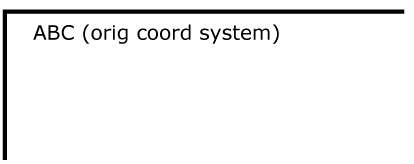Example NewCoordSys establishes a new user coordinate system by specifying transform="translate(50,50)" on the third 'g' element below. The new user coordinate system has its origin at location (50,50) in the original coordinate system. The result of this transformation is that the coordinate (30,30) in the new user coordinate system gets mapped to coordinate (80,80) in the original coordinate system (i.e., the coordinates have been translated by 50 units in X and 50 units in Y).

```<?xml version="1.0" standalone="no"?>
<!DOCTYPE svg PUBLIC "-//W3C//DTD SVG 20000802//EN"
"http://www.w3.org/TR/2000/CR-SVG-20000802/DTD/svg-20000802.dtd">
<svg width="400px" height="150px">
<desc>Example NewCoordSys - New user coordinate system</desc>
<g style="fill:none; stroke:black; stroke-width:3">
<!-- Draw the axes of the original coordinate system -->
<line x1="0" y1="1.5" x2="400" y2="1.5" />
<line x1="1.5" y1="0" x2="1.5" y2="150" />
</g>
<g>
<text x="30" y="30" style="font-size:20 font-family:Verdana">
ABC (orig coord system)
</text>
</g>
<!-- Establish a new coordinate system, which is
shifted (i.e., translated) from the initial coordinate
system by 50 user units along each axis. -->
<g transform="translate(50,50)">
<g style="fill:none; stroke:red; stroke-width:3">
<!-- Draw lines of length 50 user units along
the axes of the new coordinate system -->
<line x1="0" y1="0" x2="50" y2="0" style="stroke:red"/>
<line x1="0" y1="0" x2="0" y2="50" />
</g>
<text x="30" y="30" style="font-size:20 font-family:Verdana">
ABC (translated coord system)
</text>
</g>
</svg>
```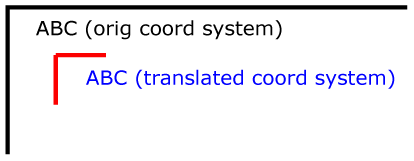Example RotateScale illustrates simple rotate and scale transformations. The example defines two new coordinate systems:

• one which is the result of a translation by 50 units in X and 30 units in Y, followed by a rotation of 30 degrees
• another which is the result of a translation by 200 units in X and 40 units in Y, followed by a scale transformation of 1.5.
```<?xml version="1.0" standalone="no"?>
<!DOCTYPE svg PUBLIC "-//W3C//DTD SVG 20000802//EN"
"http://www.w3.org/TR/2000/CR-SVG-20000802/DTD/svg-20000802.dtd">
<svg width="400px" height="120px">
<desc>Example RotateScale - Rotate and scale transforms</desc>
<g style="fill:none; stroke:black; stroke-width:3">
<!-- Draw the axes of the original coordinate system -->
<line x1="0" y1="1.5" x2="400" y2="1.5" />
<line x1="1.5" y1="0" x2="1.5" y2="120" />
</g>
<!-- Establish a new coordinate system whose origin is at (50,30)
in the initial coord. system and which is rotated by 30 degrees. -->
<g transform="translate(50,30)">
<g transform="rotate(30)">
<g style="fill:none; stroke:red; stroke-width:3">
<line x1="0" y1="0" x2="50" y2="0" />
<line x1="0" y1="0" x2="0" y2="50" />
</g>
<text x="0" y="0" style="font-size:20; font-family:Verdana; fill:blue">
ABC (rotate)
</text>
</g>
</g>
<!-- Establish a new coordinate system whose origin is at (200,40)
in the initial coord. system and which is scaled by 1.5. -->
<g transform="translate(200,40)">
<g transform="scale(1.5)">
<g style="fill:none; stroke:red; stroke-width:3">
<line x1="0" y1="0" x2="50" y2="0" />
<line x1="0" y1="0" x2="0" y2="50" />
</g>
<text x="0" y="0" style="font-size:20; font-family:Verdana; fill:blue">
ABC (scale)
</text>
</g>
</g>
</svg>
```Example Skew defines two coordinate systems which are skewed relative to the origin coordinate system.

```<?xml version="1.0" standalone="no"?>
<!DOCTYPE svg PUBLIC "-//W3C//DTD SVG 20000802//EN"
"http://www.w3.org/TR/2000/CR-SVG-20000802/DTD/svg-20000802.dtd">
<svg width="400px" height="120px">
<desc>Example Skew - Show effects of skewX and skewY</desc>
<g style="fill:none; stroke:black; stroke-width:3">
<!-- Draw the axes of the original coordinate system -->
<line x1="0" y1="1.5" x2="400" y2="1.5" />
<line x1="1.5" y1="0" x2="1.5" y2="120" />
</g>
<!-- Establish a new coordinate system whose origin is at (30,30)
in the initial coord. system and which is skewed in X by 30 degrees. -->
<g transform="translate(30,30)">
<g transform="skewX(30)">
<g style="fill:none; stroke:red; stroke-width:3">
<line x1="0" y1="0" x2="50" y2="0" />
<line x1="0" y1="0" x2="0" y2="50" />
</g>
<text x="0" y="0" style="font-size:20; font-family:Verdana; fill:blue">
ABC (skewX)
</text>
</g>
</g>
<!-- Establish a new coordinate system whose origin is at (200,30)
in the initial coord. system and which is skewed in Y by 30 degrees. -->
<g transform="translate(200,30)">
<g transform="skewY(30)">
<g style="fill:none; stroke:red; stroke-width:3">
<line x1="0" y1="0" x2="50" y2="0" />
<line x1="0" y1="0" x2="0" y2="50" />
</g>
<text x="0" y="0" style="font-size:20; font-family:Verdana; fill:blue">
ABC (skewY)
</text>
</g>
</g>
</svg>
```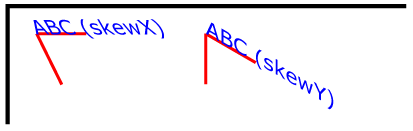Mathematically, all transformations can be represented as 3x3 transformation matrices of the following form: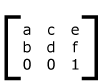Since only six values are used in the above 3x3 matrix, a transformation matrix is also expressed as a vector: [a b c d e f].

Transformations map coordinates and lengths from a new coordinate system into a previous coordinate system:Simple transformations are represented in matrix form as follows:

• Translation is equivalent to the matrix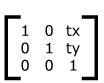or [1 0 0 1 tx ty], where tx and ty are the distances to translate coordinates in X and Y, respectively.

• Scaling is equivalent to the matrix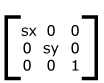or [sx 0 0 sy 0 0]. One unit in the X and Y directions in the new coordinate system equals sx and sy units in the previous coordinate system, respectively.

• Rotation about the origin is equivalent to the matrix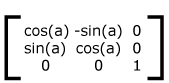or [cos(a) sin(a) -sin(a) cos(a) 0 0], which has the effect of rotating the coordinate system axes by angle a.

• A skew transformation along the x-axis is equivalent to the matrix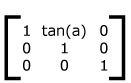or [1 0 tan(a) 1 0 0], which has the effect of skewing X coordinates by angle a.

• A skew transformation along the y-axis is equivalent to the matrixor [1 tan(a) 0 1 0 0], which has the effect of skewing Y coordinates by angle a.

## 7.5 Nested transformations

Transformations can be nested to any level. The effect of nested transformations is to post-multiply (i.e., concatenate) the subsequent transformation matrices onto previously defined transformations: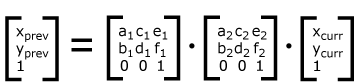For each given element, the accumulation of all transformations that have been defined on the given element and all of its ancestors up to and including the element which established the current viewport (usually, the 'svg' element which is the most immediate ancestor to the given element) is called the current transformation matrix or CTM. The CTM thus represents the mapping of current user coordinates to viewport coordinates: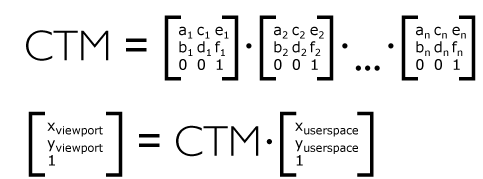Example Nested illustrates nested transformations.

```<?xml version="1.0" standalone="no"?>
<!DOCTYPE svg PUBLIC "-//W3C//DTD SVG 20000802//EN"
"http://www.w3.org/TR/2000/CR-SVG-20000802/DTD/svg-20000802.dtd">
<svg width="400px" height="150px">
<desc>Example Nested - Nested transformations</desc>
<g style="fill:none; stroke:black; stroke-width:3">
<!-- Draw the axes of the original coordinate system -->
<line x1="0" y1="1.5" x2="400" y2="1.5" />
<line x1="1.5" y1="0" x2="1.5" y2="150" />
</g>
<!-- First, a translate -->
<g transform="translate(50.90)">
<g style="fill:none; stroke:red; stroke-width:3">
<line x1="0" y1="0" x2="50" y2="0" />
<line x1="0" y1="0" x2="0" y2="50" />
</g>
<text x="0" y="0" style="font-size:16; font-family:Verdana">
....Translate(1)
</text>
<!-- Second, a rotate -->
<g transform="rotate(-45)">
<g style="fill:none; stroke:green; stroke-width:3">
<line x1="0" y1="0" x2="50" y2="0" />
<line x1="0" y1="0" x2="0" y2="50" />
</g>
<text x="0" y="0" style="font-size:16; font-family:Verdana">
....Rotate(2)
</text>
<!-- Third, another translate -->
<g transform="translate(130,160)">
<g style="fill:none; stroke:blue; stroke-width:3">
<line x1="0" y1="0" x2="50" y2="0" />
<line x1="0" y1="0" x2="0" y2="50" />
</g>
<text x="0" y="0" style="font-size:16; font-family:Verdana">
....Translate(3)
</text>
</g>
</g>
</g>
</svg>
```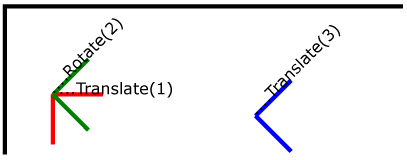In the example above, the CTM within the third nested transformation (i.e., the transform="translate(130,160)") consists of the concatenation of the three transformations, as follows: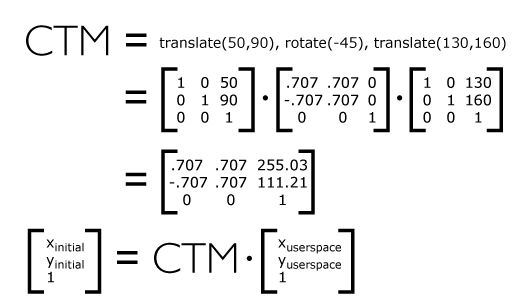## 7.6 The transform attribute

The value of the transform attribute is a <transform-list>, which is defined as a list of transform definitions, which are applied in the order provided. The individual transform definitions are separated by whitespace and/or a comma. The available types of transform definitions include:

• matrix(<a> <b> <c> <d> <e> <f>), which specifies a transformation in the form of a transformation matrix of six values. matrix(a,b,c,d,e,f) is equivalent to applying the transformation matrix [a b c d e f].

• translate(<tx> [<ty>]), which specifies a translation by tx and ty.

• scale(<sx> [<sy>]), which specifies a scale operation by sx and sy. If <sy> is not provided, it is assumed to be equal to <sx>.

• rotate(<rotate-angle> [<cx> <cy>]), which specifies a rotation by <rotate-angle> degrees about a given point.
If optional parameters <cx> and <cy> are not supplied, the rotate is about the origin of the current user coordinate system. The operation corresponds to the matrix [cos(a) sin(a) -sin(a) cos(a) 0 0].
If optional parameters <cx> and <cy> are supplied, the rotate is about the point (<cx>, <cy>). The operation represents the equivalent of the following specification: translate(<cx>, <cy>) rotate(<rotate-angle>) translate(-<cx>, -<cy>).

• skewX(<skew-angle>), which specifies a skew transformation along the x-axis.

• skewY(<skew-angle>), which specifies a skew transformation along the y-axis.

All numeric values are real <number>s.

If a list of transforms is provided, then the net effect is as if each transform had been specified separately in the order provided. For example,

```<g transform="translate(-10,-20) scale(2) rotate(45) translate(5,10)">
<!-- graphics elements go here -->
</g>
```

is functionally equivalent to:

```<g transform="translate(-10,-20)">
<g transform="scale(2)">
<g transform="rotate(45)">
<g transform="translate(5,10)">
<!-- graphics elements go here -->
</g>
</g>
</g>
</g>
```

The transform attribute is applied to an element before processing any other coordinate or length values supplied for that element. In the element

```<rect x="10" y="10" width="20" height="20" transform="scale(2)"/>
```

the x, y, width and height values are processed after the current coordinate system has been scaled uniformly by a factor of 2 by the transform attribute. Attributes x, y, width and height (and any other attributes or properties) are treated as values in the new user coordinate system, not the previous user coordinate system. Thus, the above 'rect' element is functionally equivalent to:

```<g transform="scale(2)">
<rect x="10" y="10" width="20" height="20"/>
</g>
```

The following is the Backus-Naur Form (BNF) for values for the transform attribute. The following notation is used:

• *: 0 or more
• +: 1 or more
• ?: 0 or 1
• (): grouping
• |: separates alternatives
• double quotes surround literals
```transform-list:
wsp* transforms? wsp*

transforms:
transform
| transform comma-wsp+ transforms

transform:
matrix
| translate
| scale
| rotate
| skewX
| skewY

matrix:
"matrix" wsp* "(" wsp*
number comma-wsp
number comma-wsp
number comma-wsp
number comma-wsp
number comma-wsp
number wsp* ")"

translate:
"translate" wsp* "(" wsp* number ( comma-wsp number )? wsp* ")"

scale:
"scale" wsp* "(" wsp* number ( comma-wsp number )? wsp* ")"

rotate:
"rotate" wsp* "(" wsp* number ( comma-wsp number comma-wsp number )? wsp* ")"

skewX:
"skewX" wsp* "(" wsp* number wsp* ")"

skewY:
"skewY" wsp* "(" wsp* number wsp* ")"

number:
sign? integer-constant
| sign? floating-point-constant

comma-wsp:
(wsp+ comma? wsp*) | (comma wsp*)

comma:
","

integer-constant:
digit-sequence

floating-point-constant:
fractional-constant exponent?
| digit-sequence exponent

fractional-constant:
digit-sequence? "." digit-sequence
| digit-sequence "."

exponent:
( "e" | "E" ) sign? digit-sequence

sign:
"+" | "-"

digit-sequence:
digit
| digit digit-sequence

digit:
"0" | "1" | "2" | "3" | "4" | "5" | "6" | "7" | "8" | "9"

wsp:
(#x20 | #x9 | #xD | #xA)
```

For the transform attribute:

Animatable: yes.

See the 'animateTransform' element for information on animating transformations.

## 7.7 The viewBox attribute

It is often desirable to specify that a given set of graphics stretch to fit a particular container element. The viewBox attribute provides this capability.

All elements that establish a new viewport (see elements that establish viewports) have attribute viewBox. The value of the viewBox attribute is a list of four numbers <min-x>, <min-y>, <width> and <height>, separated by whitespace and/or a comma, which specify a rectangle in user space which should be mapped to the bounds of the viewport established by the given element, taking into account attribute preserveAspectRatio. If specified, an additional transformation is applied to all descendants of the given element to achieve the specified effect.

A negative value for <width> or <height> is an error (see Error processing). A value of zero disables rendering of the element.

Example ViewBox illustrates the use of the viewBox attribute on the outermost 'svg' element to specify that the SVG content should stretch to fit bounds of the viewport.

```<?xml version="1.0" standalone="no"?>
<!DOCTYPE svg PUBLIC "-//W3C//DTD SVG 20000802//EN"
"http://www.w3.org/TR/2000/CR-SVG-20000802/DTD/svg-20000802.dtd">
<svg width="300px" height="200px"
viewBox="0 0 1500 1000" preserveAspectRatio="none" >
<desc>Example ViewBox - uses the viewBox
attribute to automatically create an initial user coordinate
system which causes the graphic to scale to fit into the
viewport no matter what size the viewport is.</desc>

<!-- This rectangle goes from (0,0) to (1500,1000) in user space.
Because of the viewBox attribute above,
the rectangle will end up filling the entire area
reserved for the SVG content. -->
<rect x="0" y="0" width="1500" height="1000" style="fill:yellow" />

<!-- A large, red triangle -->
<path style="fill:red" d="M 750,100 L 250,900 L 1250,900 z"/>

<!-- A text string that spans most of the viewport -->
<text x="100" y="600" style="font-size:150; font-family:Verdana">
Stretch to fit
</text>
</svg>
```
Example ViewBox
Rendered into
viewport with
width=300px,
height=200px
Rendered into
viewport with
width=150px,
height=200px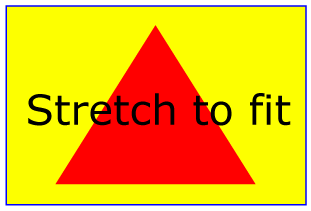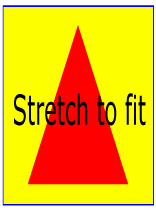The effect of the viewBox attribute is that the user agent automatically supplies the appropriate transformation matrix to map the specified rectangle in user space to the bounds of the viewport. To achieve the effect of the example on the left, with viewport dimensions of 300 by 200 pixels, the user agent needs to automatically insert a transformation which scales both X and Y by 0.2. The effect is equivalent to having a viewport of size 300px by 200px and the following supplemental transformation in the document, as follows:

```<?xml version="1.0" standalone="no"?>
<!DOCTYPE svg PUBLIC "-//W3C//DTD SVG 20000802//EN"
"http://www.w3.org/TR/2000/CR-SVG-20000802/DTD/svg-20000802.dtd">
<svg width="300px" height="200px">

<g transform="scale(0.2)">

<!-- Rest of document goes here -->

</g>
</svg>
```
To achieve the effect of the example on the right, with viewport dimensions of 150 by 200 pixels, the user agent needs to automatically insert a transformation which scales X by 0.1 and Y by 0.2. The effect is equivalent to having a viewport of size 150px by 200px and the following supplemental transformation in the document, as follows:
```<?xml version="1.0" standalone="no"?>
<!DOCTYPE svg PUBLIC "-//W3C//DTD SVG 20000802//EN"
"http://www.w3.org/TR/2000/CR-SVG-20000802/DTD/svg-20000802.dtd">
<svg width="150px" height="200px">

<g transform="scale(0.1 0.2)">

<!-- Rest of document goes here -->

</g>
</svg>
```

(Note: in some cases the user agent will need to supply a translate transformation in addition to a scale transformation. For example, on an outermost 'svg', a translate transformation will be needed if the viewBox attributes specifies values other than zero for <min-x> or <min-y>.)

For the viewBox attribute:

Animatable: yes.

## 7.8 The preserveAspectRatio attribute

In some cases when using the viewBox attribute, it is desirable that the graphics stretch to fit non-uniformly to take up the entire viewport. In other cases when using the viewBox attribute, it is desirable that uniform scaling be used for the purposes of preserving the aspect ratio of the graphics.

Attribute preserveAspectRatio="<align> [<meetOrSlice>]", which is available for all elements that establish a new viewport (see elements that establish viewports), indicates whether or not to force uniform scaling. preserveAspectRatio only applies when a value has been provided for viewBox on the same element. If attribute viewBox is not provided, then preserveAspectRatio is ignored.

The <align> parameter indicates whether to force uniform scaling and, if so, the alignment method to use in case the aspect ratio of the viewBox doesn't match the aspect ratio of the viewport. The <align> parameter must be one of the following strings:

• none - Do not force uniform scaling. Scale the graphic content of the given element non-uniformly if necessary such that the element's bounding box exactly matches the viewport rectangle.
(Note: if <align> is none, then the optional <meetOrSlice> value is ignored.)
• xMinYMin - Force uniform scaling.
Align the <min-x> of the element's viewBox with the smallest X value of the viewport.
Align the <min-y> of the element's viewBox with the smallest Y value of the viewport.
• xMidYMin - Force uniform scaling.
Align the midpoint X value of the element's viewBox with the midpoint X value of the viewport.
Align the <min-y> of the element's viewBox with the smallest Y value of the viewport.
• xMaxYMin - Force uniform scaling.
Align the <min-x>+<width> of the element's viewBox with the maximum X value of the viewport.
Align the <min-y> of the element's viewBox with the smallest Y value of the viewport.
• xMinYMid - Force uniform scaling.
Align the <min-x> of the element's viewBox with the smallest X value of the viewport.
Align the midpoint Y value of the element's viewBox with the midpoint Y value of the viewport.
• xMidYMid (the default) - Force uniform scaling.
Align the midpoint X value of the element's viewBox with the midpoint X value of the viewport.
Align the midpoint Y value of the element's viewBox with the midpoint Y value of the viewport.
• xMaxYMid - Force uniform scaling.
Align the <min-x>+<width> of the element's viewBox with the maximum X value of the viewport.
Align the midpoint Y value of the element's viewBox with the midpoint Y value of the viewport.
• xMinYMax - Force uniform scaling.
Align the <min-x> of the element's viewBox with the smallest X value of the viewport.
Align the <min-y>+<height> of the element's viewBox with the maximum Y value of the viewport.
• xMidYMax - Force uniform scaling.
Align the midpoint X value of the element's viewBox with the midpoint X value of the viewport.
Align the <min-y>+<height> of the element's viewBox with the maximum Y value of the viewport.
• xMaxYMax - Force uniform scaling.
Align the <min-x>+<width> of the element's viewBox with the maximum X value of the viewport.
Align the <min-y>+<height> of the element's viewBox with the maximum Y value of the viewport.

The <meetOrSlice> parameter is optional and, if provided, is separated from the <align> value by one or more spaces and then must be one of the following strings:

• meet (the default) - Scale the graphic such that:
• aspect ratio is preserved
• the entire viewBox is visible within the viewport
• the viewBox is scaled up as much as possible, while still meeting the other criteria
In this case, if the aspect ratio of the graphic does not match the viewport, some of the viewport will extend beyond the bounds of the viewBox (i.e., the area into which the viewBox will draw will be smaller than the viewport).
• slice - Scale the graphic such that:
• aspect ratio is preserved
• the entire viewport is covered by the viewBox
• the viewBox is scaled down as much as possible, while still meeting the other criteria
In this case, if the aspect ratio of the viewBox does not match the viewport, some of the viewBox will extend beyond the bounds of the viewport (i.e., the area into which the viewBox will draw is larger than the viewport).

Example PreserveAspectRatio illustrates the various options on preserveAspectRatio. To save space, XML entities have been defined for the three repeated graphic objects, the rectangle with the smile inside and the outlines of the two rectangles which have the same dimensions as the target viewports. The example creates several new viewports by including 'svg' sub-elements embedded inside the outermost 'svg' element (see Establishing a new viewport). The smile is drawing the text string ":)" rotated 90 degrees.

```<?xml version="1.0" standalone="no"?>
<!DOCTYPE svg PUBLIC "-//W3C//DTD SVG 20000802//EN"
"http://www.w3.org/TR/2000/CR-SVG-20000802/DTD/svg-20000802.dtd" [
<!ENTITY Smile "
<rect x='.5' y='.5' width='29' height='39' style='fill:yellow; stroke:red'/>
<g transform='rotate(90)'>
<text x='10' y='10' style='font-family:Verdana;
font-weight:bold; font-size:14'>:)</text>
</g>">
<!ENTITY Viewport1 "<rect x='.5' y='.5' width='49' height='29'
style='fill:none; stroke:blue'/>">
<!ENTITY Viewport2 "<rect x='.5' y='.5' width='29' height='59'
style='fill:none; stroke:blue'/>">
]>
<svg width="480px" height="270px" style="font-family:Verdana; font-size:8">
<desc>Example PreserveAspectRatio - demonstrate available options</desc>
<text x="10" y="30">SVG to fit</text>
<g transform="translate(20,40)">&Smile;</g>
<text x="10" y="110">Viewport 1</text>
<g transform="translate(10,120)">&Viewport1;</g>
<text x="10" y="180">Viewport 2</text>
<g transform="translate(20,190)">&Viewport2;</g>
<text x="100" y="30">--------------- meet ---------------</text>
<g transform="translate(100,60)"><text y="-10">xMin*</text>&Viewport1;
<svg preserveAspectRatio="xMinYMin meet" viewBox="0 0 30 40"
width="50" height="30">&Smile;</svg></g>
<g transform="translate(170,60)"><text y="-10">xMid*</text>&Viewport1;
<svg preserveAspectRatio="xMidYMid meet" viewBox="0 0 30 40"
width="50" height="30">&Smile;</svg></g>
<g transform="translate(240,60)"><text y="-10">xMax*</text>&Viewport1;
<svg preserveAspectRatio="xMaxYMax meet" viewBox="0 0 30 40"
width="50" height="30">&Smile;</svg></g>
<text x="330" y="30">---------- meet ----------</text>
<g transform="translate(330,60)"><text y="-10">*YMin</text>&Viewport2;
<svg preserveAspectRatio="xMinYMin meet" viewBox="0 0 30 40"
width="30" height="60">&Smile;</svg></g>
<g transform="translate(380,60)"><text y="-10">*YMid</text>&Viewport2;
<svg preserveAspectRatio="xMidYMid meet" viewBox="0 0 30 40"
width="30" height="60">&Smile;</svg></g>
<g transform="translate(430,60)"><text y="-10">*YMax</text>&Viewport2;
<svg preserveAspectRatio="xMaxYMax meet" viewBox="0 0 30 40"
width="30" height="60">&Smile;</svg></g>
<text x="100" y="160">---------- slice ----------</text>
<g transform="translate(100,190)"><text y="-10">xMin*</text>&Viewport2;
<svg preserveAspectRatio="xMinYMin slice" viewBox="0 0 30 40"
width="30" height="60">&Smile;</svg></g>
<g transform="translate(150,190)"><text y="-10">xMid*</text>&Viewport2;
<svg preserveAspectRatio="xMidYMid slice" viewBox="0 0 30 40"
width="30" height="60">&Smile;</svg></g>
<g transform="translate(200,190)"><text y="-10">xMax*</text>&Viewport2;
<svg preserveAspectRatio="xMaxYMax slice" viewBox="0 0 30 40"
width="30" height="60">&Smile;</svg></g>
<text x="270" y="160">--------------- slice ---------------</text>
<g transform="translate(270,190)"><text y="-10">*YMin</text>&Viewport1;
<svg preserveAspectRatio="xMinYMin slice" viewBox="0 0 30 40"
width="50" height="30">&Smile;</svg></g>
<g transform="translate(340,190)"><text y="-10">*YMid</text>&Viewport1;
<svg preserveAspectRatio="xMidYMid slice" viewBox="0 0 30 40"
width="50" height="30">&Smile;</svg></g>
<g transform="translate(410,190)"><text y="-10">*YMax</text>&Viewport1;
<svg preserveAspectRatio="xMaxYMax slice" viewBox="0 0 30 40"
width="50" height="30">&Smile;</svg></g>
</svg>
```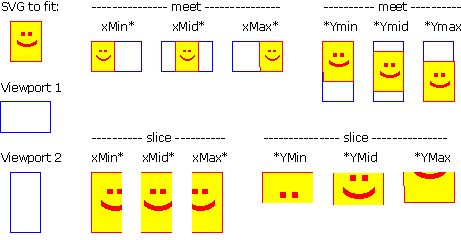For the preserveAspectRatio attribute:

Animatable: yes.

## 7.9 Establishing a new viewport

At any point in an SVG drawing, you can establish a new viewport into which all contained graphics is drawn by including an 'svg' element inside SVG content. By establishing a new viewport, you also implicitly establish a new viewport coordinate system, a new user coordinate system, new meanings for many of the unit identifiers and, potentially, a new clipping path.

The bounds of the new viewport are defined by the x, y, width and height attributes on the element establishing the new viewport, such as an 'svg' element. Both the new viewport coordinate system and the new user coordinate system have their origins at (x, y), where x and y represent the value of the corresponding attributes on the element establishing the viewport. The orientation of the new viewport coordinate system and the new user coordinate system correspond to the orientation of the current user coordinate system for the element establishing the viewport. A single unit in the new viewport coordinate system and the new user coordinate system are the same size as a single unit in the current user coordinate system for the element establishing the viewport.

Here is an example:

```<?xml version="1.0" standalone="no"?>
<!DOCTYPE svg PUBLIC "-//W3C//DTD SVG 20000802//EN"
"http://www.w3.org/TR/2000/CR-SVG-20000802/DTD/svg-20000802.dtd">
<svg width="4in" height="3in">
<desc>This SVG drawing embeds another one,
thus establishing a new viewport
</desc>
<!-- The following statement establishing a new viewport
and renders SVG drawing B into that viewport -->
<svg x="25%" y="25%" width="50%" height="50%">
<!-- drawing B goes here -->
</svg>
</svg>
```

For an extensive example of creating new viewports, see Example PreserveAspectRatio.

In addition to the 'svg' element, any elements which are defined such that they are processed in the same manner as an 'svg' element also establish a new viewport. In particular:

• A 'symbol' element define new viewports whenever they are instanced by a 'use' element.
• An 'image' element that references an SVG file will result in the establishment of a temporary new viewport since the referenced resource by definition will have an 'svg' element.
• A 'foreignObject' element creates a new viewport for rendering the content that is within the element.

Whether a new viewport also establishes a new additional clipping path is determined by the value of the 'overflow' property on the element which establishes the new viewport. If a clipping path is created to correspond to the new viewport, the clipping path's geometry is determined by the value of the 'clip' property. Also, see Clip to viewport vs. clip to viewBox.

## 7.10 Units

All coordinates and lengths in SVG can be specified with or without a unit identifier.

If a coordinate or length value is a number without a unit identifier (e.g., "25"), then the given coordinate or length is assumed to be in user units (i.e., a value in user space). For example:

```<text style="font-size: 50">Text size is 50 user units</text>
```

If coordinate or length value is a number following by a unit identifier (e.g., "25cm" or "15em"), then the given coordinate represents either an absolute length in viewport units or a relative length (i.e., a value relative to some other distance measurement). The list of unit identifiers in SVG matches the list of unit identifiers in CSS: em, ex, px, pt, pc, cm, mm, in and percentages.

As in CSS, the em and ex unit identifiers are relative to the current font's font-size and x-height, respectively.

When the various absolute unit identifiers (i.e., px, pt, pc, cm, mm, in) are used, the coordinate and length values represent values within the viewport coordinate system and do not change their meaning as transformations alter the current coordinate system. Thus, "12pt" can be made to represent exactly 12 points on the actual visual medium even if the user coordinate system has been scaled.

Example AbsoluteUnits illustrates how absolute units do not scale even when transformations are applied.

```<?xml version="1.0" standalone="no"?>
<!DOCTYPE svg PUBLIC "-//W3C//DTD SVG 20000802//EN"
"http://www.w3.org/TR/2000/CR-SVG-20000802/DTD/svg-20000802.dtd">
<svg width="450px" height="150px" style="font-family:Verdana">
<desc>Example AbsoluteUnits - Absolute units and coordinate transformations
</desc>
<!-- Draw an outline of the drawing in blue -->
<rect x="1" y="1" width="448" height="148" style="fill:none; stroke:blue"/>

<!-- The following two text elements will both draw with a
font height of 12 pixels -->
<text x="20" y="25" style="font-size: 12; fill: black">
This draws 12 pixels high.
</text>
<text x="20" y="50" style="font-size: 12px; fill: red">
This draws 12 pixels high.
</text>

<!-- Now scale the coordinate system by 2. -->
<g transform="scale(2)">

<!-- The following text will actually draw 24 pixels high
because each unit in the new coordinate system equals
2 units in the previous coordinate system. -->
<text x="20" y="50" style="font-size: 12; fill:green">
This draws 24 pixels high.
</text>

<!-- The following text will actually still draw 12 pixels high
because the unit identifier has been provided. -->
<text x="20" y="62.5" style="font-size: 12px; fill:blue">
This draws 12 pixels high.
</text>
</g>
</svg>
```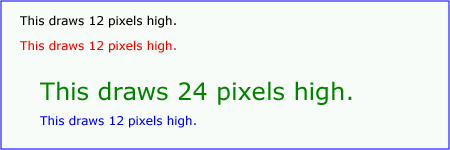When the current SVG document fragment has not yet been subject to transformations (e.g., when you have a standalone SVG document or an SVG document fragment embedded directly within a XML document styled with CSS2 or XSL), then the initial value for a px unit must be set in conformance with the rules for px units as described in [CSS2 lengths]. If the SVG implementation is part of a user agent which provides for styling XML documents using CSS2-compatible px units, then the SVG user agent should get its initial value for a px unit to match the value used for other XML styling operations.

Note that use of px units can cause inconsistent visual results on different systems; thus, px units are only recommended for situations where positioning must be aligned relative to the device pixel grid, such as when SVG content needs to align visually with other pixel-aligned XML content.

## 7.11 Redefining the meaning of unit identifiers

The process of establishing a new viewport, such as when there is an 'svg' element inside of another SVG 'svg', changes the meaning of the following unit identifiers: px, pt, pc, cm, mm, in, and % (percentages). A "pixel" (the px unit) becomes equivalent to a single unit in the user coordinate system for the given 'svg' element. The meaning of the other absolute unit identifiers (pt, pc, cm, mm, in) are determined as an appropriate multiple of a px unit using the actual size of px unit (as passed from the parent user agent to the SVG user agent). Any percentage values that are relative to the current viewport will also represent new values.

Example AbsoluteUnitsRedefined illustrates how absolute units identifiers have their meaning changed when a new viewport is established.

```<?xml version="1.0" standalone="no"?>
<!DOCTYPE svg PUBLIC "-//W3C//DTD SVG 20000802//EN"
"http://www.w3.org/TR/2000/CR-SVG-20000802/DTD/svg-20000802.dtd">
<svg width="480px" height="225px" style="font-family:Verdana">
<desc>Example AbsoluteUnitsRedefined - Transformation with establishment of a new viewport
</desc>
<!-- Draw an outline of the drawing in blue -->
<rect x="1" y="1" width="478" height="223" style="fill:none; stroke:blue"/>

<!-- The following two text elements will both draw with a
font height of 12 pixels -->
<text x="20" y="25" style="font-size: 12; fill: black">
This draws 12 pixels high.
</text>
<text x="20" y="50" style="font-size: 12px; fill: red">
This draws 12 pixels high.
</text>

<!-- This time, scale the coordinate system by 3. -->
<g transform="scale(3)">

<!-- Establish a new viewport and thus change the meaning of
some unit identifiers. -->
<svg x="0" y="25" width="160" height="50">
<!-- Draw an interior outline of the viewport in brown -->
<rect x="1" y="1" width="158" height="48"
style="fill:none; stroke:brown; stroke-width:.33333"/>

<!-- The following two text elements will both draw with a
font height of 36 screen pixels. The first text element
defines its height in user coordinates, which have been
scaled by 3. The second text element defines its height
in px units, which have been redefined to be three times
as big as screen pixels due the <svg> element establishing
a new viewport. -->
<text x="2" y="20" style="font-size: 12; fill:magenta">
This draws 36 pixels high.
</text>
<text x="2" y="40" style="font-size: 12px; fill:cyan">
This draws 36 pixels high.
</text>
</svg>
</g>
</svg>
```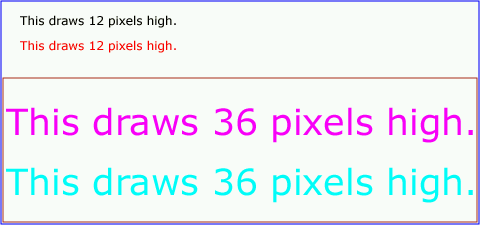## 7.12 Object bounding box units

The following elements offer the option of expressing coordinate values and lengths as fractions (and, in some cases, percentages) of the bounding box (via keyword objectBoundingBox) on a given element:

Element Attribute Effect
'linearGradient' gradientUnits="objectBoundingBox" Indicates that the attributes which specify the gradient vector (x1, y1, x2, y2) represent fractions or percentages of the bounding box of the element to which the gradient is applied.
'radialGradient' gradientUnits="objectBoundingBox" Indicates that the attributes which specify the center (cx, cy), the radius (r) and focus (fx, fy) represent fractions or percentages of the bounding box of the element to which the gradient is applied.
'pattern' patternUnits="objectBoundingBox" Indicates that the attributes which define how to tile the pattern (x, y, width, height) and that the user coordinate system for the contents of the pattern is established using the bounding box of the element to which the pattern is applied.
'clipPath' clipPathUnits="objectBoundingBox" Indicates that the user coordinate system for the contents of the 'clipPath' element is established using the bounding box of the element to which the clipping path is applied.
'mask' maskUnits="objectBoundingBox" Indicates that the user coordinate system for the contents of the 'mask' element is established using the bounding box of the element to which the mask is applied.
'filter' filterUnits="objectBoundingBox" Indicates that the attributes which define the filter effects region (x, y, width, height) represent fractions or percentages of the bounding box of the element to which the filter is applied.
'filter' primitiveUnits="objectBoundingBox" Indicates that the various length values within the filter primitives represent fractions or percentages of the bounding box of the element to which the filter is applied.

When keyword objectBoundingBox is used, then the effect is as if a supplemental transformation matrix were inserted into the list of nested transformation matrices to create a new user coordinate system.

First, the (minx,miny) and (maxx,maxy) coordinates are determined for the referencing element and all of its descendants. The values minx, miny, maxx and maxy are determined by computing the maximum extent of the shape of the element in X and Y with respect to the user coordinate system for the referencing element. The bounding box is the tightest fitting rectangle aligned with the axes of the referencing element's user coordinate system that entirely encloses the referencing element and its descendants. The bounding box is computed exclusive of any values for clipping, masking, filter effects, opacity and stroke-width. For curved shapes, the bounding box encloses all portions of the shape, not just end points. For 'text' elements, for the purposes of the bounding box calculation, each glyph is treated as a separate graphics element. The calculations assume that all glyphs occupy the full glyph cell. For example, for horizontal text, the calculations assume that each glyph extends vertically to the full ascent and descent values for the font.

Then, coordinate (0,0) in the new user coordinate system is mapped to the (minx,miny) corner of the tight bounding box within the user coordinate system of the referencing element and coordinate (1,1) in the new user coordinate system is mapped to the (maxx,maxy) corner of the tight bounding box of the referencing element. In most situations, the following transformation matrix produces the correct effect:

`[ (maxx-minx) 0 0 (maxy-miny) minx miny ]`

When percentages are used with keyword objectBoundingBox, a percentage represents the same value as the corresponding decimal value (e.g., 50% means the same as 0.5).

Keyword objectBoundingBox should not be used when the geometry of the referencing element has no width or no height, such as the case of a horizontal or vertical line, even when the line has actual thickness when viewed due to having a non-zero stroke width since stroke width is ignored for bounding box calculations. When the geometry of the referencing element has no width or height and objectBoundingBox is specified, then the given effect (e.g., a gradient or a filter) will be ignored.

## 7.13 Processing rules when using absolute unit identifiers and percentages

Even when coordinates and lengths in SVG have an absolute unit identifier or represent a percentage (see Units), these values are first mapped into user space, and then processing occurs as if the values had been specified as the corresponding value in user space.

For coordinates and lengths in SVG which have an absolute unit identifier or represent a percentage of the viewport, the values are converted into user space values as follows:

• For any x-coordinate value or width value (xValueInVPSpace) expressed using an absolute unit identifier, first convert xValueInVPSpace into viewport pixel units using the SVG user agent's standard conversion factor from pixels to real world units (e.g., millimeters) to yield xValueInVPPixels. Then transform the points (0,0) and (xValueInVPPixels,0), from viewport space to current user space using the inverse of the current transformation matrix, yielding two points in userspace Q1 and Q2. Do a distance calculation between Q1 and Q2 (sqrt((Q2x-Q1x)**2 + (Q2y-Q1y)**2)) and use that as the value for the given operation.
• For any y-coordinate value or height value (yValueInVPSpace) expressed using an absolute unit identifier, then use the same method as above, except use points (0,0) and (0,yValueInVPPixels) instead.
• For any x-coordinate value or width value (xValueInVPSpace) expressed as a percentage of the viewport, transform the points (0,0) and (percentageValue*vpWidthInPixels,0), from viewport space to current user space using the inverse of the current transformation matrix, yielding two points in userspace Q1 and Q2. Do a distance calculation between Q1 and Q2 (sqrt((Q2x-Q1x)**2 + (Q2y-Q1y)**2)) and use that as the value for the given operation.
• For any y-coordinate value or height value (yValueInVPSpace) expressed as a percentage of the viewport, then use the same method as above, except use points (0,0) and (0,percentageValue*vpHeightInPixels) instead.
• For any other length value in viewport space (lengthVPSpace), the following approach is used to give appropriate weighting to the contribution of the two dimensions of the viewport. First, convert lengthVPSpace into viewport pixel units using the SVG user agent's standard conversion factor from pixels to real world units (e.g., millimeters) to yield lengthVPPixels. Calculate the distance from (0,0) and (vpWidthInPixels,vpHeightInPixels) in viewport space using the formula: vpDiagLengthVPPixels=sqrt(vpWidthInPixels**2 + vpHeightInPixels**2). Using the inverse of the current transformation matrix, determine the points in user space (P1x,P1y) and (P2x,P2y) which correspond to the points (0,0) and (vpWidthInPixels,vpHeightInPixels) in viewport space. Calculate the distance from (P1x,P1y) and (P2x,P2y) in user space using the formula: vpDiagLengthUserSpace=sqrt((P2x-P1x)**2 + (P2y-P1y)**2)). Then, convert the original viewport-relative length into a length in user space using the formula: lengthUserSpace = lengthVPPixels * (vpDiagLengthUserSpace/vpDiagLengthVPPixels).
• If a viewport-relative percentage value is given, then use the same method as above, except calculate lengthVPPixels as lengthVPPixels=percentageValue*sqrt(vpWidthPixels**2 + vpHeightPixels**2)/sqrt(2).

Any values expressed as fractions or percentages of the current object bounding box are mapped to corresponding values in user space as follows:

• For any x-coordinate value or width value, determine the minimum and maximum x-coordinates in user space for the object bounding box (bboxXMinUserSpace and bboxXMaxUserSpace, respectively). An x-coordinate value is converted into a coordinate in user space using the formula bboxXMinUserSpace+percentageValue*(bboxXMaxUserSpace-bboxXMinUserSpace) and a width value is converted into a length in user space using the formula percentageValue*(bboxXMaxUserSpace-bboxXMinUserSpace).
• For any y-coordinate value or width value, determine the minimum and maximum y-coordinates in user space for the object bounding box (bboxYMinUserSpace and bboxYMaxUserSpace, respectively). A y-coordinate value is converted into a coordinate in user space using the formula bboxYMinUserSpace+percentageValue*(bboxYMaxUserSpace-bboxYMinUserSpace) and a height value is converted into a length in user space using the formula percentageValue*(bboxYMaxUserSpace-bboxYMinUserSpace).
• For any other length value expressed as a fraction or percentage of the current object bounding box, determine the minimum and maximum x and y coordinates in user space for the object bounding box (bboxXMinUserSpace, bboxXMaxUserSpace, bboxYMinUserSpace and bboxYMaxUserSpace), calculate bboxWidth=bboxXMaxUserSpace-bboxXMinUserSpace and bboxHeight=bboxYMaxUserSpace-bboxYMinUserSpace, and then map the fraction or percentage of the current object bounding box to a length in user space using the formula percentageValue*sqrt(bboxWidth**2 + bboxHeight**2)/sqrt(2).

## 7.14 DOM interfaces

The following interfaces are defined below: SVGPoint, SVGMatrix, SVGTransformList, SVGAnimatedTransformList, SVGTransform, SVGPreserveAspectRatio, SVGAnimatedPreserveAspectRatio.

### Interface SVGPoint

Many of the SVG DOM interfaces refer to objects of class SVGPoint. An SVGPoint is an (x,y) coordinate pair. When used in matrix operations, an SVGPoint is treated as a vector of the form:

```[x]
[y]
```

IDL Definition
```interface SVGPoint {

attribute float x;
// raises DOMException on setting
attribute float y;
// raises DOMException on setting

SVGPoint matrixTransform ( in SVGMatrix matrix );
};```

Attributes
float x
The x coordinate.
Exceptions on setting
 DOMException NO_MODIFICATION_ALLOWED_ERR: Raised when the node is readonly.
float y
The y coordinate.
Exceptions on setting
 DOMException NO_MODIFICATION_ALLOWED_ERR: Raised when the node is readonly.
Methods
matrixTransform

Applies a 2x3 matrix transformation on this SVGPoint object and returns a new, transformed SVGPoint object:

`newpoint = matrix * thispoint`
Parameters
 in SVGMatrix matrix The matrix which is to be applied to this SVGPoint object.
Return value
 SVGPoint A new SVGPoint object.
No Exceptions

### Interface SVGMatrix

Many of SVG's graphics operations utilize 2x3 matrices of the form:

```[a c e]
[b d f]```

which, when expanded into a 3x3 matrix for the purposes of matrix arithmetic, become:

```[a c e]
[b d f]
[0 0 1]```

IDL Definition
```interface SVGMatrix {

attribute float a;
// raises DOMException on setting
attribute float b;
// raises DOMException on setting
attribute float c;
// raises DOMException on setting
attribute float d;
// raises DOMException on setting
attribute float e;
// raises DOMException on setting
attribute float f;
// raises DOMException on setting

SVGMatrix multiply ( in SVGMatrix secondMatrix );
SVGMatrix inverse (  )
raises( SVGException );
SVGMatrix translate ( in float x, in float y );
SVGMatrix scale ( in float scaleFactor );
SVGMatrix scaleNonUniform ( in float scaleFactorX, in float scaleFactorY );
SVGMatrix rotate ( in float angle );
SVGMatrix rotateFromVector ( in float x, in float y )
raises( SVGException );
SVGMatrix flipX (  );
SVGMatrix flipY (  );
SVGMatrix skewX ( in float angle );
SVGMatrix skewY ( in float angle );
};```

Attributes
float a
The a component of the matrix.
Exceptions on setting
 DOMException NO_MODIFICATION_ALLOWED_ERR: Raised when the node is readonly.
float b
The b component of the matrix.
Exceptions on setting
 DOMException NO_MODIFICATION_ALLOWED_ERR: Raised when the node is readonly.
float c
The c component of the matrix.
Exceptions on setting
 DOMException NO_MODIFICATION_ALLOWED_ERR: Raised when the node is readonly.
float d
The d component of the matrix.
Exceptions on setting
 DOMException NO_MODIFICATION_ALLOWED_ERR: Raised when the node is readonly.
float e
The e component of the matrix.
Exceptions on setting
 DOMException NO_MODIFICATION_ALLOWED_ERR: Raised when the node is readonly.
float f
The f component of the matrix.
Exceptions on setting
 DOMException NO_MODIFICATION_ALLOWED_ERR: Raised when the node is readonly.
Methods
multiply
Performs matrix multiplication. This matrix is post-multiplied by another matrix, returning the resulting new matrix.
Parameters
 in SVGMatrix secondMatrix The matrix which is post-multiplied to this matrix.
Return value
 SVGMatrix The resulting matrix.
No Exceptions
inverse
Returns the inverse matrix.
No Parameters
Return value
 SVGMatrix The inverse matrix.
Exceptions
 SVGException SVG_MATRIX_NOT_INVERTABLE: Raised if this matrix is not invertable.
translate
Post-multiplies a translation transformation on the current matrix and returns the resulting matrix.
Parameters
 in float x The distance to translate along the x-axis. in float y The distance to translate along the y-axis.
Return value
 SVGMatrix The resulting matrix.
No Exceptions
scale
Post-multiplies a uniform scale transformation on the current matrix and returns the resulting matrix.
Parameters
 in float scaleFactor Scale factor in both X and Y.
Return value
 SVGMatrix The resulting matrix.
No Exceptions
scaleNonUniform
Post-multiplies a non-uniform scale transformation on the current matrix and returns the resulting matrix.
Parameters
 in float scaleFactorX Scale factor in X. in float scaleFactorY Scale factor in Y.
Return value
 SVGMatrix The resulting matrix.
No Exceptions
rotate
Post-multiplies a rotation transformation on the current matrix and returns the resulting matrix.
Parameters
 in float angle Rotation angle.
Return value
 SVGMatrix The resulting matrix.
No Exceptions
rotateFromVector
Post-multiplies a rotation transformation on the current matrix and returns the resulting matrix. The rotation angle is determined by taking (+/-) atan(y/x). The direction of the vector (x,y) determines whether the positive or negative angle value is used.
Parameters
 in float x The X coordinate of the vector (x,y). Must not be zero. in float y The Y coordinate of the vector (x,y). Must not be zero.
Return value
 SVGMatrix The resulting matrix.
Exceptions
 SVGException SVG_INVALID_VALUE_ERR: Raised if one of the parameters has an invalid value.
flipX
Post-multiplies the transformation [-1 0 0 1 0 0] and returns the resulting matrix.
No Parameters
Return value
 SVGMatrix The resulting matrix.
No Exceptions
flipY
Post-multiplies the transformation [1 0 0 -1 0 0] and returns the resulting matrix.
No Parameters
Return value
 SVGMatrix The resulting matrix.
No Exceptions
skewX
Post-multiplies a skewX transformation on the current matrix and returns the resulting matrix.
Parameters
 in float angle Skew angle.
Return value
 SVGMatrix The resulting matrix.
No Exceptions
skewY
Post-multiplies a skewY transformation on the current matrix and returns the resulting matrix.
Parameters
 in float angle Skew angle.
Return value
 SVGMatrix The resulting matrix.
No Exceptions

### Interface SVGTransformList

SVGTransformList maintains an ordered list of SVGTransform objects. The SVGTransformList and SVGTransform interfaces correspond to the various attributes which specify a set of transformations, such as the transform attribute which is available for many of SVG's elements.

The various methods inherited from SVGList, which are defined in SVGList to accept parameters and return values of type Object, must receive parameters of type SVGTransform and return values of type SVGTransform.

IDL Definition
```interface SVGTransformList : SVGList {
SVGTransform createSVGTransformFromMatrix ( in SVGMatrix matrix );
SVGTransform consolidate (  );
};```

Methods
createSVGTransformFromMatrix
Creates an SVGTransform object which is initialized to transform of type SVG_TRANSFORM_MATRIX and whose values are the given matrix.
Parameters
 in SVGMatrix matrix The matrix which defines the transformation.
Return value
 SVGTransform The returned SVGTransform object.
No Exceptions
consolidate
Consolidates the list of separate SVGTransform objects by multiplying the equivalent transformation matrices together to result in a list consisting of a single SVGTransform object of type SVG_TRANSFORM_MATRIX.
No Parameters
Return value
 SVGTransform The resulting SVGTransform object which becomes single item in the list. If the list was empty, then a value of null is returned.
No Exceptions

### Interface SVGAnimatedTransformList

Used for the various attributes which specify a set of transformations, such as the transform attribute which is available for many of SVG's elements, and which can be animated.

IDL Definition
```interface SVGAnimatedTransformList {

attribute SVGTransformList baseVal;
// raises DOMException on setting
readonly attribute SVGTransformList animVal;
};```

Attributes
SVGTransformList baseVal
The base value of the given attribute before applying any animations.
Exceptions on setting
 DOMException NO_MODIFICATION_ALLOWED_ERR: Raised when the node is readonly.
readonly SVGTransformList animVal
If the given attribute or property is being animated, contains the current animated value of the attribute or property. If the given attribute or property is not currently being animated, contains the same value as 'baseVal'.

### Interface SVGTransform

SVGTransform is the interface for one of the component transformations within a SVGTransformList; thus, a SVGTransform object corresponds to a single component (e.g., "scale(..)" or "matrix(...)") within a transform attribute specification.

IDL Definition
```interface SVGTransform {

// Transform Types
const unsigned short SVG_TRANSFORM_UNKNOWN   = 0;
const unsigned short SVG_TRANSFORM_MATRIX    = 1;
const unsigned short SVG_TRANSFORM_TRANSLATE = 2;
const unsigned short SVG_TRANSFORM_SCALE     = 3;
const unsigned short SVG_TRANSFORM_ROTATE    = 4;
const unsigned short SVG_TRANSFORM_SKEWX     = 5;
const unsigned short SVG_TRANSFORM_SKEWY     = 6;

readonly attribute unsigned short type;
readonly attribute SVGMatrix matrix;
readonly attribute float angle;

void setMatrix ( in SVGMatrix matrix );
void setTranslate ( in float tx, in float ty );
void setScale ( in float sx, in float sy );
void setRotate ( in float angle, in float cx, in float cy );
void setSkewX ( in float angle );
void setSkewY ( in float angle );
};```

Definition group Transform Types
Defined constants
 SVG_TRANSFORM_UNKNOWN The unit type is not one of predefined types. It is invalid to attempt to define a new value of this type or to attempt to switch an existing value to this type. SVG_TRANSFORM_MATRIX A "matrix(...)" transformation. SVG_TRANSFORM_TRANSLATE A "translate(...)" transformation. SVG_TRANSFORM_SCALE A "scale(...)" transformation. SVG_TRANSFORM_ROTATE A "rotate(...)" transformation. SVG_TRANSFORM_SKEWX A "skewX(...)" transformation. SVG_TRANSFORM_SKEWY A "skewY(...)" transformation.
Attributes
readonly unsigned short type
The type of the value as specified by one of the constants specified above.
readonly SVGMatrix matrix
The matrix that represents this transformation.
For SVG_TRANSFORM_MATRIX, the matrix contains the a, b, c, d, e, f values supplied by the user.
For SVG_TRANSFORM_TRANSLATE, e and f represents the translation amounts (a=1,b=0,c=0,d=1).
For SVG_TRANSFORM_SCALE, a and d represents the scale amounts (b=0,c=0,e=0,f=0).
For SVG_TRANSFORM_ROTATE, SVG_TRANSFORM_SKEWX and SVG_TRANSFORM_SKEWY, a, b, c and d represent the matrix which will result in the given transformation (e=0,f=0).
readonly float angle
A convenience attribute for SVG_TRANSFORM_ROTATE, SVG_TRANSFORM_SKEWX and SVG_TRANSFORM_SKEWY. It holds the angle that was specified.
For SVG_TRANSFORM_MATRIX, SVG_TRANSFORM_TRANSLATE and SVG_TRANSFORM_SCALE, angle will be zero.
Methods
setMatrix
Sets the transform type to SVG_TRANSFORM_MATRIX, with parameter matrix defining the new transformation.
Parameters
 in SVGMatrix matrix The new matrix for the transformation.
No Return Value
No Exceptions
setTranslate
Sets the transform type to SVG_TRANSFORM_TRANSLATE, with parameters tx and ty defining the translation amounts.
Parameters
 in float tx The translation amount in X. in float ty The translation amount in Y.
No Return Value
No Exceptions
setScale
Sets the transform type to SVG_TRANSFORM_SCALE, with parameters sx and sy defining the scale amounts.
Parameters
 in float sx The scale factor in X. in float sy The scale factor in Y.
No Return Value
No Exceptions
setRotate
Sets the transform type to SVG_TRANSFORM_ROTATE, with parameter angle defining the rotation angle and parameters cx and cy defining the optional centre of rotation.
Parameters
 in float angle The rotation angle. in float cx The x coordinate of centre of rotation. in float cy The y coordinate of centre of rotation.
No Return Value
No Exceptions
setSkewX
Sets the transform type to SVG_TRANSFORM_SKEWX, with parameter angle defining the amount of skew.
Parameters
 in float angle The skew angle.
No Return Value
No Exceptions
setSkewY
Sets the transform type to SVG_TRANSFORM_SKEWY, with parameter angle defining the amount of skew.
Parameters
 in float angle The skew angle.
No Return Value
No Exceptions

### Interface SVGPreserveAspectRatio

The SVGPreserveAspectRatio interface corresponds to the preserveAspectRatio attribute, which is available for some of SVG's elements.

IDL Definition
```interface SVGPreserveAspectRatio {

// Alignment Types
const unsigned short SVG_PRESERVEASPECTRATIO_UNKNOWN   = 0;
const unsigned short SVG_PRESERVEASPECTRATIO_NONE     = 1;
const unsigned short SVG_PRESERVEASPECTRATIO_XMINYMIN = 2;
const unsigned short SVG_PRESERVEASPECTRATIO_XMIDYMIN = 3;
const unsigned short SVG_PRESERVEASPECTRATIO_XMAXYMIN = 4;
const unsigned short SVG_PRESERVEASPECTRATIO_XMINYMID = 5;
const unsigned short SVG_PRESERVEASPECTRATIO_XMIDYMID = 6;
const unsigned short SVG_PRESERVEASPECTRATIO_XMAXYMID = 7;
const unsigned short SVG_PRESERVEASPECTRATIO_XMINYMAX = 8;
const unsigned short SVG_PRESERVEASPECTRATIO_XMIDYMAX = 9;
const unsigned short SVG_PRESERVEASPECTRATIO_XMAXYMAX = 10;
// Meet-or-slice Types
const unsigned short SVG_MEETORSLICE_UNKNOWN   = 0;
const unsigned short SVG_MEETORSLICE_MEET  = 1;
const unsigned short SVG_MEETORSLICE_SLICE = 2;

attribute unsigned short align;
// raises DOMException on setting
attribute unsigned short meetOrSlice;
// raises DOMException on setting
};```

Definition group Alignment Types
Defined constants
 SVG_PRESERVEASPECTRATIO_UNKNOWN The enumeration was set to a value that is not one of predefined types. It is invalid to attempt to define a new value of this type or to attempt to switch an existing value to this type. SVG_PRESERVEASPECTRATIO_NONE Corresponds to value 'none' for attribute preserveAspectRatio. SVG_PRESERVEASPECTRATIO_XMINYMIN Corresponds to value 'xMinYMin' for attribute preserveAspectRatio. SVG_PRESERVEASPECTRATIO_XMIDYMIN Corresponds to value 'xMidYMin' for attribute preserveAspectRatio. SVG_PRESERVEASPECTRATIO_XMAXYMIN Corresponds to value 'xMaxYMin' for attribute preserveAspectRatio. SVG_PRESERVEASPECTRATIO_XMINYMID Corresponds to value 'xMinYMid' for attribute preserveAspectRatio. SVG_PRESERVEASPECTRATIO_XMIDYMID Corresponds to value 'xMidYMid' for attribute preserveAspectRatio. SVG_PRESERVEASPECTRATIO_XMAXYMID Corresponds to value 'xMaxYMid' for attribute preserveAspectRatio. SVG_PRESERVEASPECTRATIO_XMINYMAX Corresponds to value 'xMinYMax' for attribute preserveAspectRatio. SVG_PRESERVEASPECTRATIO_XMIDYMAX Corresponds to value 'xMidYMax' for attribute preserveAspectRatio. SVG_PRESERVEASPECTRATIO_XMAXYMAX Corresponds to value 'xMaxYMax' for attribute preserveAspectRatio.
Definition group Meet-or-slice Types
Defined constants
 SVG_MEETORSLICE_UNKNOWN The enumeration was set to a value that is not one of predefined types. It is invalid to attempt to define a new value of this type or to attempt to switch an existing value to this type. SVG_MEETORSLICE_MEET Corresponds to value 'meet' for attribute preserveAspectRatio. SVG_MEETORSLICE_SLICE Corresponds to value 'slice' for attribute preserveAspectRatio.
Attributes
unsigned short align
The type of the alignment value as specified by one of the constants specified above.
Exceptions on setting
 DOMException NO_MODIFICATION_ALLOWED_ERR: Raised when the node is readonly.
unsigned short meetOrSlice
The type of the meet-or-slice value as specified by one of the constants specified above.
Exceptions on setting
 DOMException NO_MODIFICATION_ALLOWED_ERR: Raised when the node is readonly.

### Interface SVGAnimatedPreserveAspectRatio

Used for attributes of type SVGPreserveAspectRatio which can be animated.

IDL Definition
```interface SVGAnimatedPreserveAspectRatio {

attribute SVGPreserveAspectRatio baseVal;
// raises DOMException on setting
readonly attribute SVGPreserveAspectRatio animVal;
};```

Attributes
SVGPreserveAspectRatio baseVal
The base value of the given attribute before applying any animations.
Exceptions on setting
 DOMException NO_MODIFICATION_ALLOWED_ERR: Raised when the node is readonly.
readonly SVGPreserveAspectRatio animVal
If the given attribute or property is being animated, contains the current animated value of the attribute or property. If the given attribute or property is not currently being animated, contains the same value as 'baseVal'.